# EWD 3 Training Course Part 23: Traversing a Range using DocumentNode Objects

IT Consultant, Developer & Director/Founder at M/Gateway Developments Ltd
Aug. 30, 2016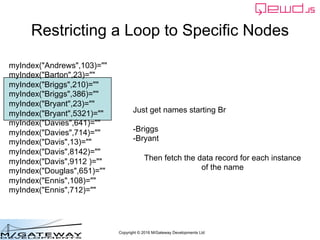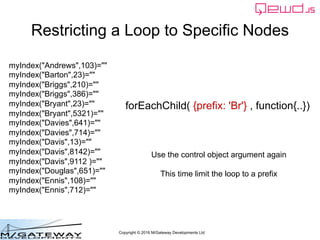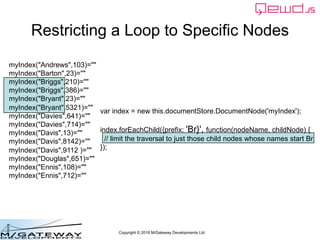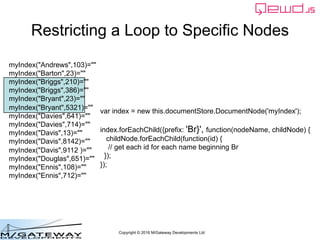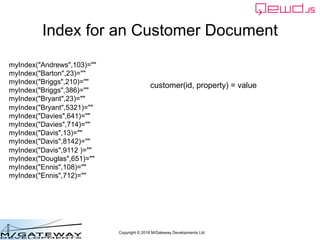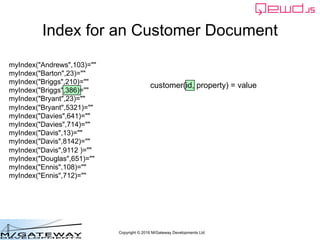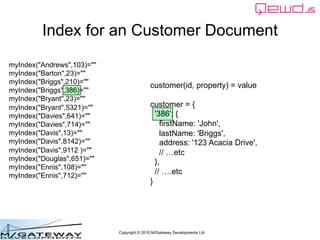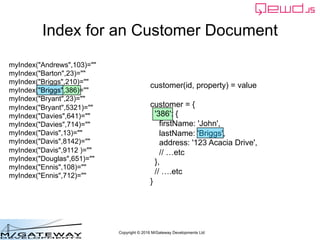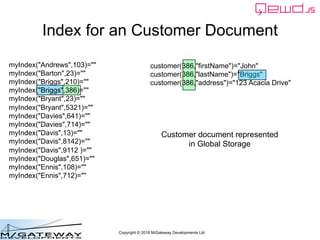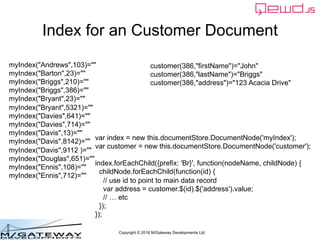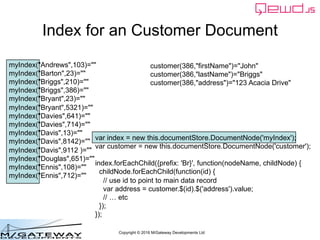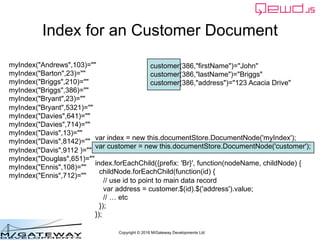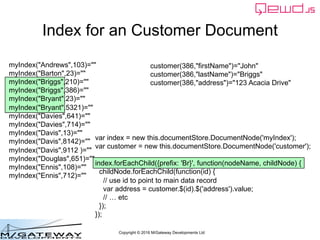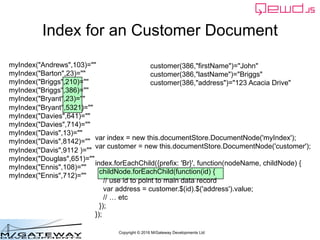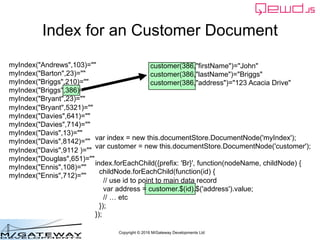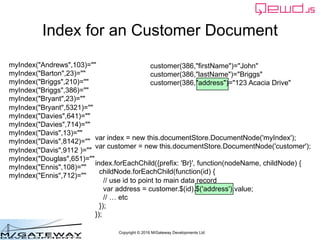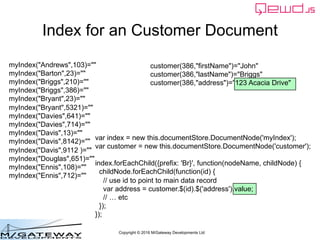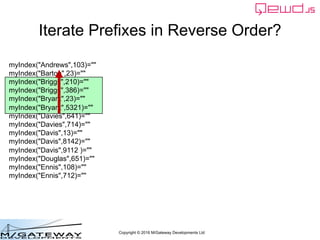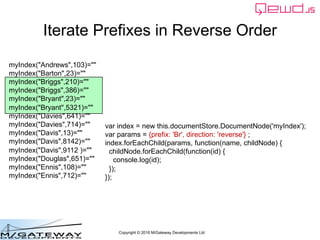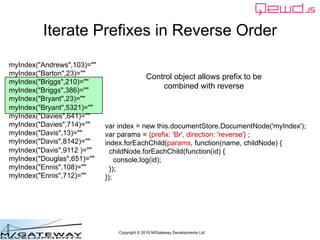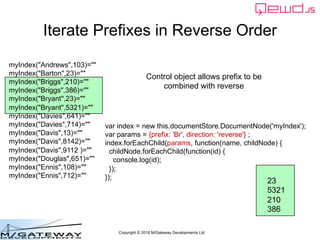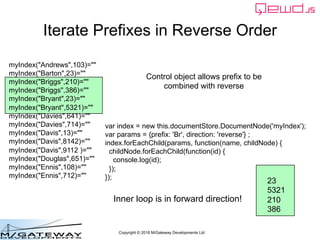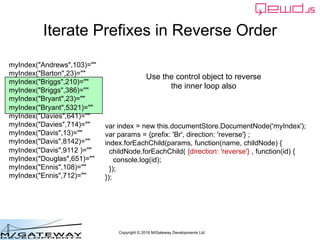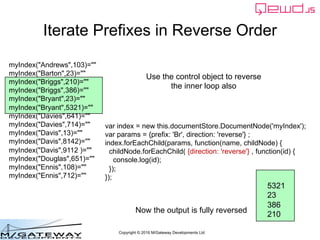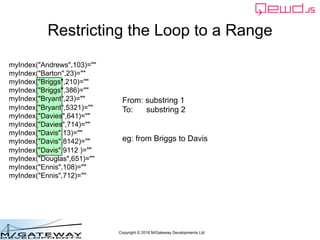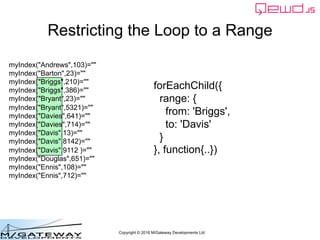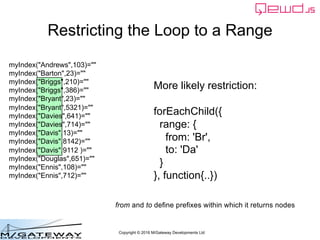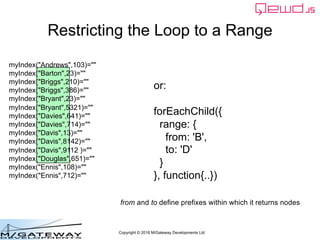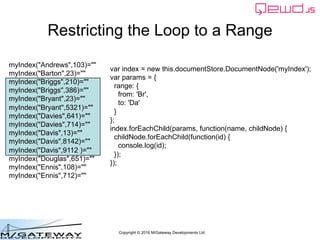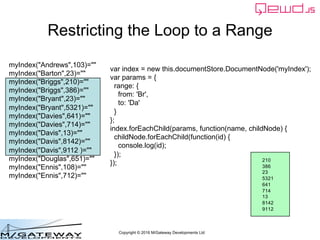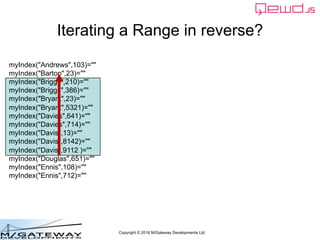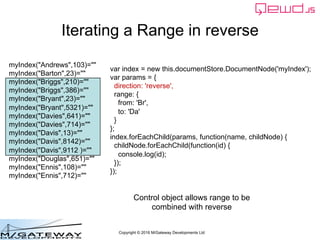1 of 35

### EWD 3 Training Course Part 23: Traversing a Range using DocumentNode Objects

• 1. Copyright © 2016 M/Gateway Developments Ltd EWD 3 Training Course Part 23 JavaScript Abstraction of Global Storage: (d) Traversing a Range of Nodes Rob Tweed Director, M/Gateway Developments Ltd Twitter: @rtweed
• 2. Copyright © 2016 M/Gateway Developments Ltd myIndex("Andrews",103)="" myIndex("Barton",23)="" myIndex("Briggs",210)="" myIndex("Briggs",386)="" myIndex("Bryant",23)="" myIndex("Bryant",5321)="" myIndex("Davies",641)="" myIndex("Davies",714)="" myIndex("Davis",13)="" myIndex("Davis",8142)="" myIndex("Davis",9112 )="" myIndex("Douglas",651)="" myIndex("Ennis",108)="" myIndex("Ennis",712)="" Restricting a Loop to Specific Nodes
• 3. Copyright © 2016 M/Gateway Developments Ltd myIndex("Andrews",103)="" myIndex("Barton",23)="" myIndex("Briggs",210)="" myIndex("Briggs",386)="" myIndex("Bryant",23)="" myIndex("Bryant",5321)="" myIndex("Davies",641)="" myIndex("Davies",714)="" myIndex("Davis",13)="" myIndex("Davis",8142)="" myIndex("Davis",9112 )="" myIndex("Douglas",651)="" myIndex("Ennis",108)="" myIndex("Ennis",712)="" Restricting a Loop to Specific Nodes Just get names starting Br -Briggs -Bryant Then fetch the data record for each instance of the name
• 4. Copyright © 2016 M/Gateway Developments Ltd myIndex("Andrews",103)="" myIndex("Barton",23)="" myIndex("Briggs",210)="" myIndex("Briggs",386)="" myIndex("Bryant",23)="" myIndex("Bryant",5321)="" myIndex("Davies",641)="" myIndex("Davies",714)="" myIndex("Davis",13)="" myIndex("Davis",8142)="" myIndex("Davis",9112 )="" myIndex("Douglas",651)="" myIndex("Ennis",108)="" myIndex("Ennis",712)="" Restricting a Loop to Specific Nodes forEachChild( {prefix: 'Br'} , function{..}) Use the control object argument again This time limit the loop to a prefix
• 5. Copyright © 2016 M/Gateway Developments Ltd myIndex("Andrews",103)="" myIndex("Barton",23)="" myIndex("Briggs",210)="" myIndex("Briggs",386)="" myIndex("Bryant",23)="" myIndex("Bryant",5321)="" myIndex("Davies",641)="" myIndex("Davies",714)="" myIndex("Davis",13)="" myIndex("Davis",8142)="" myIndex("Davis",9112 )="" myIndex("Douglas",651)="" myIndex("Ennis",108)="" myIndex("Ennis",712)="" Restricting a Loop to Specific Nodes var index = new this.documentStore.DocumentNode('myIndex'); index.forEachChild({prefix: 'Br}', function(nodeName, childNode) { // limit the traversal to just those child nodes whose names start Br });
• 6. Copyright © 2016 M/Gateway Developments Ltd myIndex("Andrews",103)="" myIndex("Barton",23)="" myIndex("Briggs",210)="" myIndex("Briggs",386)="" myIndex("Bryant",23)="" myIndex("Bryant",5321)="" myIndex("Davies",641)="" myIndex("Davies",714)="" myIndex("Davis",13)="" myIndex("Davis",8142)="" myIndex("Davis",9112 )="" myIndex("Douglas",651)="" myIndex("Ennis",108)="" myIndex("Ennis",712)="" Restricting a Loop to Specific Nodes var index = new this.documentStore.DocumentNode('myIndex'); index.forEachChild({prefix: 'Br}', function(nodeName, childNode) { childNode.forEachChild(function(id) { // get each id for each name beginning Br }); });
• 7. Copyright © 2016 M/Gateway Developments Ltd myIndex("Andrews",103)="" myIndex("Barton",23)="" myIndex("Briggs",210)="" myIndex("Briggs",386)="" myIndex("Bryant",23)="" myIndex("Bryant",5321)="" myIndex("Davies",641)="" myIndex("Davies",714)="" myIndex("Davis",13)="" myIndex("Davis",8142)="" myIndex("Davis",9112 )="" myIndex("Douglas",651)="" myIndex("Ennis",108)="" myIndex("Ennis",712)="" Restricting a Loop to Specific Nodes var index = new this.documentStore.DocumentNode('myIndex'); index.forEachChild({prefix: 'Br}', function(nodeName, childNode) { childNode.forEachChild(function(id) { console.log(id); }); }); 210 386 23 5321
• 8. Copyright © 2016 M/Gateway Developments Ltd myIndex("Andrews",103)="" myIndex("Barton",23)="" myIndex("Briggs",210)="" myIndex("Briggs",386)="" myIndex("Bryant",23)="" myIndex("Bryant",5321)="" myIndex("Davies",641)="" myIndex("Davies",714)="" myIndex("Davis",13)="" myIndex("Davis",8142)="" myIndex("Davis",9112 )="" myIndex("Douglas",651)="" myIndex("Ennis",108)="" myIndex("Ennis",712)="" Index for an Customer Document customer(id, property) = value
• 9. Copyright © 2016 M/Gateway Developments Ltd myIndex("Andrews",103)="" myIndex("Barton",23)="" myIndex("Briggs",210)="" myIndex("Briggs",386)="" myIndex("Bryant",23)="" myIndex("Bryant",5321)="" myIndex("Davies",641)="" myIndex("Davies",714)="" myIndex("Davis",13)="" myIndex("Davis",8142)="" myIndex("Davis",9112 )="" myIndex("Douglas",651)="" myIndex("Ennis",108)="" myIndex("Ennis",712)="" Index for an Customer Document customer(id, property) = value
• 10. Copyright © 2016 M/Gateway Developments Ltd myIndex("Andrews",103)="" myIndex("Barton",23)="" myIndex("Briggs",210)="" myIndex("Briggs",386)="" myIndex("Bryant",23)="" myIndex("Bryant",5321)="" myIndex("Davies",641)="" myIndex("Davies",714)="" myIndex("Davis",13)="" myIndex("Davis",8142)="" myIndex("Davis",9112 )="" myIndex("Douglas",651)="" myIndex("Ennis",108)="" myIndex("Ennis",712)="" Index for an Customer Document customer(id, property) = value customer = { '386': { firstName: 'John', lastName: 'Briggs', address: '123 Acacia Drive', // …etc }, // ….etc }
• 11. Copyright © 2016 M/Gateway Developments Ltd myIndex("Andrews",103)="" myIndex("Barton",23)="" myIndex("Briggs",210)="" myIndex("Briggs",386)="" myIndex("Bryant",23)="" myIndex("Bryant",5321)="" myIndex("Davies",641)="" myIndex("Davies",714)="" myIndex("Davis",13)="" myIndex("Davis",8142)="" myIndex("Davis",9112 )="" myIndex("Douglas",651)="" myIndex("Ennis",108)="" myIndex("Ennis",712)="" Index for an Customer Document customer(id, property) = value customer = { '386': { firstName: 'John', lastName: 'Briggs', address: '123 Acacia Drive', // …etc }, // ….etc }
• 12. Copyright © 2016 M/Gateway Developments Ltd myIndex("Andrews",103)="" myIndex("Barton",23)="" myIndex("Briggs",210)="" myIndex("Briggs",386)="" myIndex("Bryant",23)="" myIndex("Bryant",5321)="" myIndex("Davies",641)="" myIndex("Davies",714)="" myIndex("Davis",13)="" myIndex("Davis",8142)="" myIndex("Davis",9112 )="" myIndex("Douglas",651)="" myIndex("Ennis",108)="" myIndex("Ennis",712)="" Index for an Customer Document customer(386,"firstName")="John" customer(386,"lastName")="Briggs" customer(386,"address")="123 Acacia Drive" Customer document represented in Global Storage
• 13. Copyright © 2016 M/Gateway Developments Ltd myIndex("Andrews",103)="" myIndex("Barton",23)="" myIndex("Briggs",210)="" myIndex("Briggs",386)="" myIndex("Bryant",23)="" myIndex("Bryant",5321)="" myIndex("Davies",641)="" myIndex("Davies",714)="" myIndex("Davis",13)="" myIndex("Davis",8142)="" myIndex("Davis",9112 )="" myIndex("Douglas",651)="" myIndex("Ennis",108)="" myIndex("Ennis",712)="" Index for an Customer Document customer(386,"firstName")="John" customer(386,"lastName")="Briggs" customer(386,"address")="123 Acacia Drive" var index = new this.documentStore.DocumentNode('myIndex'); var customer = new this.documentStore.DocumentNode('customer'); index.forEachChild({prefix: 'Br}', function(nodeName, childNode) { childNode.forEachChild(function(id) { // use id to point to main data record var address = customer.\$(id).\$('address').value; // … etc }); });
• 14. Copyright © 2016 M/Gateway Developments Ltd myIndex("Andrews",103)="" myIndex("Barton",23)="" myIndex("Briggs",210)="" myIndex("Briggs",386)="" myIndex("Bryant",23)="" myIndex("Bryant",5321)="" myIndex("Davies",641)="" myIndex("Davies",714)="" myIndex("Davis",13)="" myIndex("Davis",8142)="" myIndex("Davis",9112 )="" myIndex("Douglas",651)="" myIndex("Ennis",108)="" myIndex("Ennis",712)="" Index for an Customer Document customer(386,"firstName")="John" customer(386,"lastName")="Briggs" customer(386,"address")="123 Acacia Drive" var index = new this.documentStore.DocumentNode('myIndex'); var customer = new this.documentStore.DocumentNode('customer'); index.forEachChild({prefix: 'Br}', function(nodeName, childNode) { childNode.forEachChild(function(id) { // use id to point to main data record var address = customer.\$(id).\$('address').value; // … etc }); });
• 15. Copyright © 2016 M/Gateway Developments Ltd myIndex("Andrews",103)="" myIndex("Barton",23)="" myIndex("Briggs",210)="" myIndex("Briggs",386)="" myIndex("Bryant",23)="" myIndex("Bryant",5321)="" myIndex("Davies",641)="" myIndex("Davies",714)="" myIndex("Davis",13)="" myIndex("Davis",8142)="" myIndex("Davis",9112 )="" myIndex("Douglas",651)="" myIndex("Ennis",108)="" myIndex("Ennis",712)="" Index for an Customer Document customer(386,"firstName")="John" customer(386,"lastName")="Briggs" customer(386,"address")="123 Acacia Drive" var index = new this.documentStore.DocumentNode('myIndex'); var customer = new this.documentStore.DocumentNode('customer'); index.forEachChild({prefix: 'Br}', function(nodeName, childNode) { childNode.forEachChild(function(id) { // use id to point to main data record var address = customer.\$(id).\$('address').value; // … etc }); });
• 16. Copyright © 2016 M/Gateway Developments Ltd myIndex("Andrews",103)="" myIndex("Barton",23)="" myIndex("Briggs",210)="" myIndex("Briggs",386)="" myIndex("Bryant",23)="" myIndex("Bryant",5321)="" myIndex("Davies",641)="" myIndex("Davies",714)="" myIndex("Davis",13)="" myIndex("Davis",8142)="" myIndex("Davis",9112 )="" myIndex("Douglas",651)="" myIndex("Ennis",108)="" myIndex("Ennis",712)="" Index for an Customer Document customer(386,"firstName")="John" customer(386,"lastName")="Briggs" customer(386,"address")="123 Acacia Drive" var index = new this.documentStore.DocumentNode('myIndex'); var customer = new this.documentStore.DocumentNode('customer'); index.forEachChild({prefix: 'Br}', function(nodeName, childNode) { childNode.forEachChild(function(id) { // use id to point to main data record var address = customer.\$(id).\$('address').value; // … etc }); });
• 17. Copyright © 2016 M/Gateway Developments Ltd myIndex("Andrews",103)="" myIndex("Barton",23)="" myIndex("Briggs",210)="" myIndex("Briggs",386)="" myIndex("Bryant",23)="" myIndex("Bryant",5321)="" myIndex("Davies",641)="" myIndex("Davies",714)="" myIndex("Davis",13)="" myIndex("Davis",8142)="" myIndex("Davis",9112 )="" myIndex("Douglas",651)="" myIndex("Ennis",108)="" myIndex("Ennis",712)="" Index for an Customer Document customer(386,"firstName")="John" customer(386,"lastName")="Briggs" customer(386,"address")="123 Acacia Drive" var index = new this.documentStore.DocumentNode('myIndex'); var customer = new this.documentStore.DocumentNode('customer'); index.forEachChild({prefix: 'Br}', function(nodeName, childNode) { childNode.forEachChild(function(id) { // use id to point to main data record var address = customer.\$(id).\$('address').value; // … etc }); });
• 18. Copyright © 2016 M/Gateway Developments Ltd myIndex("Andrews",103)="" myIndex("Barton",23)="" myIndex("Briggs",210)="" myIndex("Briggs",386)="" myIndex("Bryant",23)="" myIndex("Bryant",5321)="" myIndex("Davies",641)="" myIndex("Davies",714)="" myIndex("Davis",13)="" myIndex("Davis",8142)="" myIndex("Davis",9112 )="" myIndex("Douglas",651)="" myIndex("Ennis",108)="" myIndex("Ennis",712)="" Index for an Customer Document customer(386,"firstName")="John" customer(386,"lastName")="Briggs" customer(386,"address")="123 Acacia Drive" var index = new this.documentStore.DocumentNode('myIndex'); var customer = new this.documentStore.DocumentNode('customer'); index.forEachChild({prefix: 'Br}', function(nodeName, childNode) { childNode.forEachChild(function(id) { // use id to point to main data record var address = customer.\$(id).\$('address').value; // … etc }); });
• 19. Copyright © 2016 M/Gateway Developments Ltd myIndex("Andrews",103)="" myIndex("Barton",23)="" myIndex("Briggs",210)="" myIndex("Briggs",386)="" myIndex("Bryant",23)="" myIndex("Bryant",5321)="" myIndex("Davies",641)="" myIndex("Davies",714)="" myIndex("Davis",13)="" myIndex("Davis",8142)="" myIndex("Davis",9112 )="" myIndex("Douglas",651)="" myIndex("Ennis",108)="" myIndex("Ennis",712)="" Index for an Customer Document customer(386,"firstName")="John" customer(386,"lastName")="Briggs" customer(386,"address")="123 Acacia Drive" var index = new this.documentStore.DocumentNode('myIndex'); var customer = new this.documentStore.DocumentNode('customer'); index.forEachChild({prefix: 'Br}', function(nodeName, childNode) { childNode.forEachChild(function(id) { // use id to point to main data record var address = customer.\$(id).\$('address').value; // … etc }); });
• 20. Copyright © 2016 M/Gateway Developments Ltd myIndex("Andrews",103)="" myIndex("Barton",23)="" myIndex("Briggs",210)="" myIndex("Briggs",386)="" myIndex("Bryant",23)="" myIndex("Bryant",5321)="" myIndex("Davies",641)="" myIndex("Davies",714)="" myIndex("Davis",13)="" myIndex("Davis",8142)="" myIndex("Davis",9112 )="" myIndex("Douglas",651)="" myIndex("Ennis",108)="" myIndex("Ennis",712)="" Index for an Customer Document customer(386,"firstName")="John" customer(386,"lastName")="Briggs" customer(386,"address")="123 Acacia Drive" var index = new this.documentStore.DocumentNode('myIndex'); var customer = new this.documentStore.DocumentNode('customer'); index.forEachChild({prefix: 'Br}', function(nodeName, childNode) { childNode.forEachChild(function(id) { // use id to point to main data record var address = customer.\$(id).\$('address').value; // … etc }); });
• 21. Copyright © 2016 M/Gateway Developments Ltd myIndex("Andrews",103)="" myIndex("Barton",23)="" myIndex("Briggs",210)="" myIndex("Briggs",386)="" myIndex("Bryant",23)="" myIndex("Bryant",5321)="" myIndex("Davies",641)="" myIndex("Davies",714)="" myIndex("Davis",13)="" myIndex("Davis",8142)="" myIndex("Davis",9112 )="" myIndex("Douglas",651)="" myIndex("Ennis",108)="" myIndex("Ennis",712)="" Iterate Prefixes in Reverse Order?
• 22. Copyright © 2016 M/Gateway Developments Ltd myIndex("Andrews",103)="" myIndex("Barton",23)="" myIndex("Briggs",210)="" myIndex("Briggs",386)="" myIndex("Bryant",23)="" myIndex("Bryant",5321)="" myIndex("Davies",641)="" myIndex("Davies",714)="" myIndex("Davis",13)="" myIndex("Davis",8142)="" myIndex("Davis",9112 )="" myIndex("Douglas",651)="" myIndex("Ennis",108)="" myIndex("Ennis",712)="" Iterate Prefixes in Reverse Order var index = new this.documentStore.DocumentNode('myIndex'); var params = {prefix: 'Br', direction: 'reverse'} ; index.forEachChild(params, function(name, childNode) { childNode.forEachChild(function(id) { console.log(id); }); });
• 23. Copyright © 2016 M/Gateway Developments Ltd myIndex("Andrews",103)="" myIndex("Barton",23)="" myIndex("Briggs",210)="" myIndex("Briggs",386)="" myIndex("Bryant",23)="" myIndex("Bryant",5321)="" myIndex("Davies",641)="" myIndex("Davies",714)="" myIndex("Davis",13)="" myIndex("Davis",8142)="" myIndex("Davis",9112 )="" myIndex("Douglas",651)="" myIndex("Ennis",108)="" myIndex("Ennis",712)="" Iterate Prefixes in Reverse Order var index = new this.documentStore.DocumentNode('myIndex'); var params = {prefix: 'Br', direction: 'reverse'} ; index.forEachChild(params, function(name, childNode) { childNode.forEachChild(function(id) { console.log(id); }); }); Control object allows prefix to be combined with reverse
• 24. Copyright © 2016 M/Gateway Developments Ltd myIndex("Andrews",103)="" myIndex("Barton",23)="" myIndex("Briggs",210)="" myIndex("Briggs",386)="" myIndex("Bryant",23)="" myIndex("Bryant",5321)="" myIndex("Davies",641)="" myIndex("Davies",714)="" myIndex("Davis",13)="" myIndex("Davis",8142)="" myIndex("Davis",9112 )="" myIndex("Douglas",651)="" myIndex("Ennis",108)="" myIndex("Ennis",712)="" Iterate Prefixes in Reverse Order var index = new this.documentStore.DocumentNode('myIndex'); var params = {prefix: 'Br', direction: 'reverse'} ; index.forEachChild(params, function(name, childNode) { childNode.forEachChild(function(id) { console.log(id); }); }); Control object allows prefix to be combined with reverse 23 5321 210 386
• 25. Copyright © 2016 M/Gateway Developments Ltd myIndex("Andrews",103)="" myIndex("Barton",23)="" myIndex("Briggs",210)="" myIndex("Briggs",386)="" myIndex("Bryant",23)="" myIndex("Bryant",5321)="" myIndex("Davies",641)="" myIndex("Davies",714)="" myIndex("Davis",13)="" myIndex("Davis",8142)="" myIndex("Davis",9112 )="" myIndex("Douglas",651)="" myIndex("Ennis",108)="" myIndex("Ennis",712)="" Iterate Prefixes in Reverse Order var index = new this.documentStore.DocumentNode('myIndex'); var params = {prefix: 'Br', direction: 'reverse'} ; index.forEachChild(params, function(name, childNode) { childNode.forEachChild(function(id) { console.log(id); }); }); Control object allows prefix to be combined with reverse 23 5321 210 386 Inner loop is in forward direction!
• 26. Copyright © 2016 M/Gateway Developments Ltd myIndex("Andrews",103)="" myIndex("Barton",23)="" myIndex("Briggs",210)="" myIndex("Briggs",386)="" myIndex("Bryant",23)="" myIndex("Bryant",5321)="" myIndex("Davies",641)="" myIndex("Davies",714)="" myIndex("Davis",13)="" myIndex("Davis",8142)="" myIndex("Davis",9112 )="" myIndex("Douglas",651)="" myIndex("Ennis",108)="" myIndex("Ennis",712)="" Iterate Prefixes in Reverse Order var index = new this.documentStore.DocumentNode('myIndex'); var params = {prefix: 'Br', direction: 'reverse'} ; index.forEachChild(params, function(name, childNode) { childNode.forEachChild( {direction: 'reverse'} , function(id) { console.log(id); }); }); Use the control object to reverse the inner loop also
• 27. Copyright © 2016 M/Gateway Developments Ltd myIndex("Andrews",103)="" myIndex("Barton",23)="" myIndex("Briggs",210)="" myIndex("Briggs",386)="" myIndex("Bryant",23)="" myIndex("Bryant",5321)="" myIndex("Davies",641)="" myIndex("Davies",714)="" myIndex("Davis",13)="" myIndex("Davis",8142)="" myIndex("Davis",9112 )="" myIndex("Douglas",651)="" myIndex("Ennis",108)="" myIndex("Ennis",712)="" Iterate Prefixes in Reverse Order var index = new this.documentStore.DocumentNode('myIndex'); var params = {prefix: 'Br', direction: 'reverse'} ; index.forEachChild(params, function(name, childNode) { childNode.forEachChild( {direction: 'reverse'} , function(id) { console.log(id); }); }); Use the control object to reverse the inner loop also 5321 23 386 210 Now the output is fully reversed
• 28. Copyright © 2016 M/Gateway Developments Ltd myIndex("Andrews",103)="" myIndex("Barton",23)="" myIndex("Briggs",210)="" myIndex("Briggs",386)="" myIndex("Bryant",23)="" myIndex("Bryant",5321)="" myIndex("Davies",641)="" myIndex("Davies",714)="" myIndex("Davis",13)="" myIndex("Davis",8142)="" myIndex("Davis",9112 )="" myIndex("Douglas",651)="" myIndex("Ennis",108)="" myIndex("Ennis",712)="" Restricting the Loop to a Range From: substring 1 To: substring 2 eg: from Briggs to Davis
• 29. Copyright © 2016 M/Gateway Developments Ltd myIndex("Andrews",103)="" myIndex("Barton",23)="" myIndex("Briggs",210)="" myIndex("Briggs",386)="" myIndex("Bryant",23)="" myIndex("Bryant",5321)="" myIndex("Davies",641)="" myIndex("Davies",714)="" myIndex("Davis",13)="" myIndex("Davis",8142)="" myIndex("Davis",9112 )="" myIndex("Douglas",651)="" myIndex("Ennis",108)="" myIndex("Ennis",712)="" Restricting the Loop to a Range forEachChild({ range: { from: 'Briggs', to: 'Davis' } }, function{..})
• 30. Copyright © 2016 M/Gateway Developments Ltd myIndex("Andrews",103)="" myIndex("Barton",23)="" myIndex("Briggs",210)="" myIndex("Briggs",386)="" myIndex("Bryant",23)="" myIndex("Bryant",5321)="" myIndex("Davies",641)="" myIndex("Davies",714)="" myIndex("Davis",13)="" myIndex("Davis",8142)="" myIndex("Davis",9112 )="" myIndex("Douglas",651)="" myIndex("Ennis",108)="" myIndex("Ennis",712)="" Restricting the Loop to a Range More likely restriction: forEachChild({ range: { from: 'Br', to: 'Da' } }, function{..}) from and to define prefixes within which it returns nodes
• 31. Copyright © 2016 M/Gateway Developments Ltd myIndex("Andrews",103)="" myIndex("Barton",23)="" myIndex("Briggs",210)="" myIndex("Briggs",386)="" myIndex("Bryant",23)="" myIndex("Bryant",5321)="" myIndex("Davies",641)="" myIndex("Davies",714)="" myIndex("Davis",13)="" myIndex("Davis",8142)="" myIndex("Davis",9112 )="" myIndex("Douglas",651)="" myIndex("Ennis",108)="" myIndex("Ennis",712)="" Restricting the Loop to a Range or: forEachChild({ range: { from: 'B', to: 'D' } }, function{..}) from and to define prefixes within which it returns nodes
• 32. Copyright © 2016 M/Gateway Developments Ltd myIndex("Andrews",103)="" myIndex("Barton",23)="" myIndex("Briggs",210)="" myIndex("Briggs",386)="" myIndex("Bryant",23)="" myIndex("Bryant",5321)="" myIndex("Davies",641)="" myIndex("Davies",714)="" myIndex("Davis",13)="" myIndex("Davis",8142)="" myIndex("Davis",9112 )="" myIndex("Douglas",651)="" myIndex("Ennis",108)="" myIndex("Ennis",712)="" Restricting the Loop to a Range var index = new this.documentStore.DocumentNode('myIndex'); var params = { range: { from: 'Br', to: 'Da' } }; index.forEachChild(params, function(name, childNode) { childNode.forEachChild(function(id) { console.log(id); }); });
• 33. Copyright © 2016 M/Gateway Developments Ltd myIndex("Andrews",103)="" myIndex("Barton",23)="" myIndex("Briggs",210)="" myIndex("Briggs",386)="" myIndex("Bryant",23)="" myIndex("Bryant",5321)="" myIndex("Davies",641)="" myIndex("Davies",714)="" myIndex("Davis",13)="" myIndex("Davis",8142)="" myIndex("Davis",9112 )="" myIndex("Douglas",651)="" myIndex("Ennis",108)="" myIndex("Ennis",712)="" Restricting the Loop to a Range var index = new this.documentStore.DocumentNode('myIndex'); var params = { range: { from: 'Br', to: 'Da' } }; index.forEachChild(params, function(name, childNode) { childNode.forEachChild(function(id) { console.log(id); }); }); 210 386 23 5321 641 714 13 8142 9112
• 34. Copyright © 2016 M/Gateway Developments Ltd myIndex("Andrews",103)="" myIndex("Barton",23)="" myIndex("Briggs",210)="" myIndex("Briggs",386)="" myIndex("Bryant",23)="" myIndex("Bryant",5321)="" myIndex("Davies",641)="" myIndex("Davies",714)="" myIndex("Davis",13)="" myIndex("Davis",8142)="" myIndex("Davis",9112 )="" myIndex("Douglas",651)="" myIndex("Ennis",108)="" myIndex("Ennis",712)="" Iterating a Range in reverse?
• 35. Copyright © 2016 M/Gateway Developments Ltd myIndex("Andrews",103)="" myIndex("Barton",23)="" myIndex("Briggs",210)="" myIndex("Briggs",386)="" myIndex("Bryant",23)="" myIndex("Bryant",5321)="" myIndex("Davies",641)="" myIndex("Davies",714)="" myIndex("Davis",13)="" myIndex("Davis",8142)="" myIndex("Davis",9112 )="" myIndex("Douglas",651)="" myIndex("Ennis",108)="" myIndex("Ennis",712)="" Iterating a Range in reverse var index = new this.documentStore.DocumentNode('myIndex'); var params = { direction: 'reverse', range: { from: 'Br', to: 'Da' } }; index.forEachChild(params, function(name, childNode) { childNode.forEachChild(function(id) { console.log(id); }); }); Control object allows range to be combined with reverse